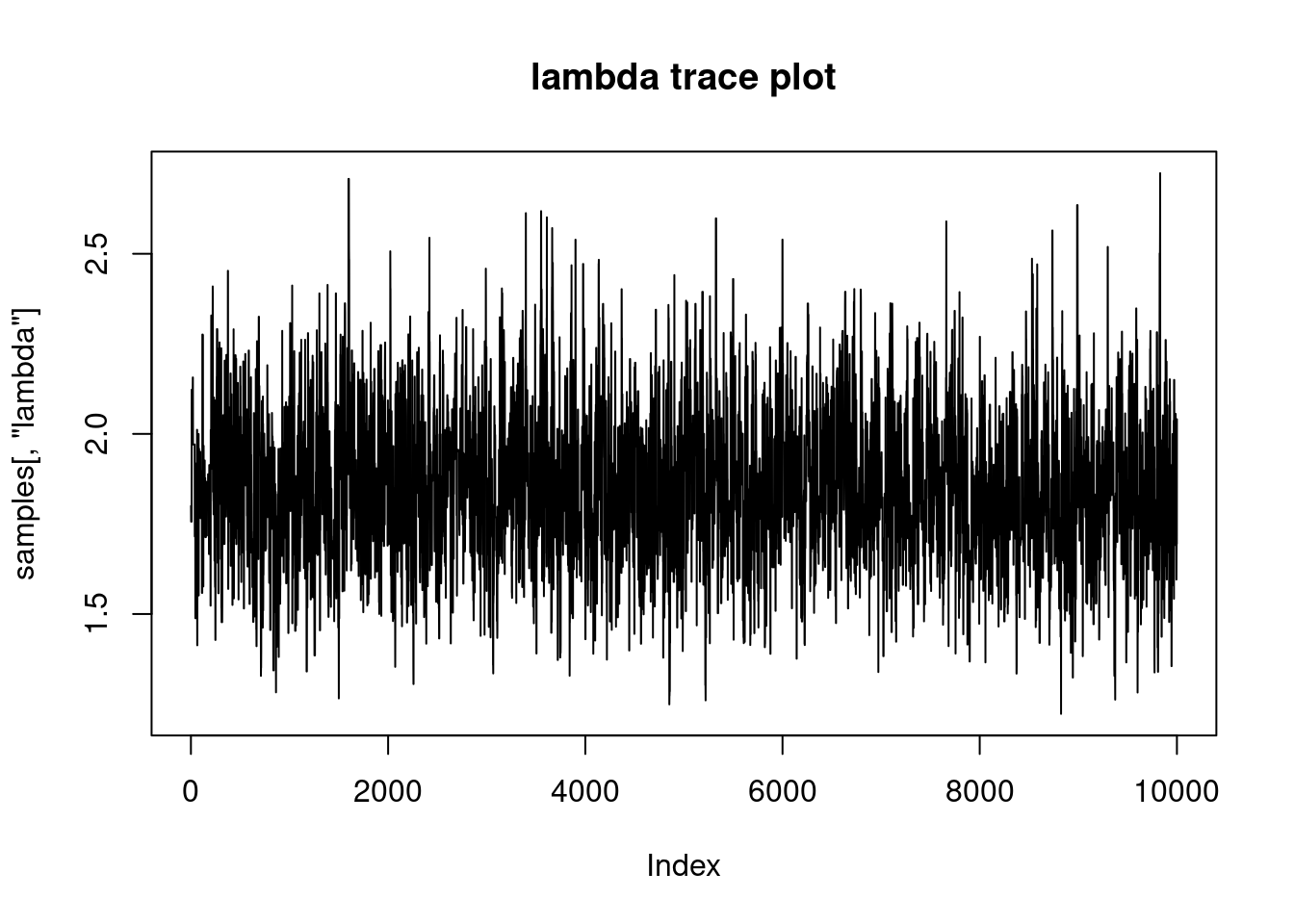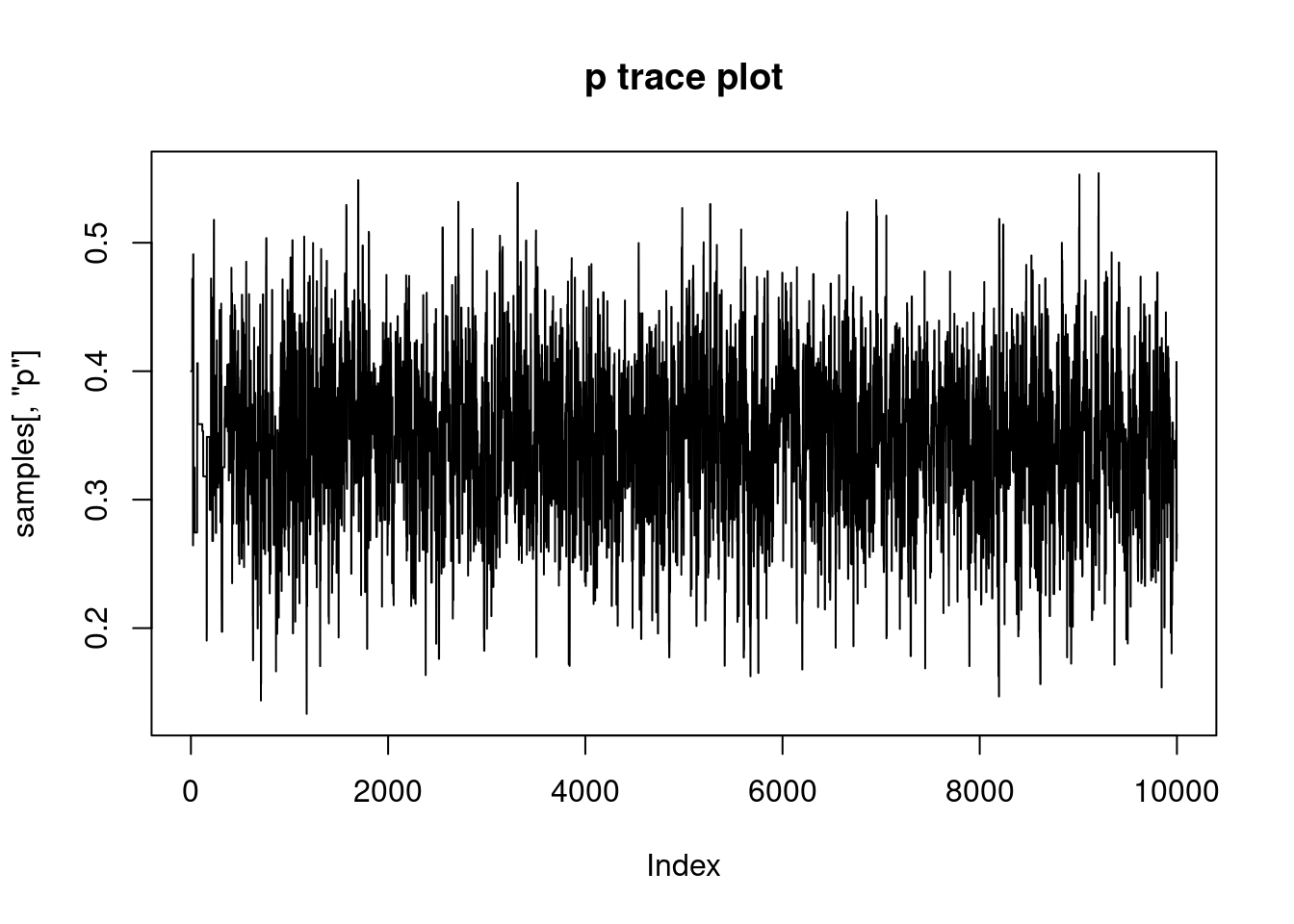# Adding a user-defined distribution to the BUGS language

In previous implementations of the BUGS language (WinBUGS, OpenBUGS, and JAGS), there is no way to extend the language with new distributions or functions (other than diving into the low-level source code). NIMBLE tries to make it easy to add distributions and functions and then to share your code with others.

## Example: zero-inflated Poisson distribution

In a variety of situations, scientists consider the possibility that some measurements will yield zeros for reasons separate from other considerations. For example, ecologists counting plants or animals of a particular species at many sites may get a zero when the species is absent but get a Poisson-distributed count when it is present. The explanatory variables they wish to consider as influencing the probability of a so-called structural zero (the species is absent) may be different from those influencing the abundance when it is present. This is done by having one parameter representing the probability of a structural zero and another parameter representing the Poisson mean. Each parameter may itself be predicted by other variables, and a zero in the data could result from either a structural zero or a Poisson sample that just happens to be zero. Similar ideas are used for zero-inflated binomial and zero-inflated negative binomial models. Hurdle models represent a subtly different approach, in which the probability of a zero is mixed with the zero-truncated probability of non-zeros.

Since such distributions were not built into earlier dialects of BUGS, users of that language have made use of a couple of indirect techniques to accomplish them. One approach is to add a Bernoulli (0/1) latent variable to the model for each observed zero. The latent variable indicates whether the corresponding data value is a structural or non-structural zero, and the MCMC must sample it. The other approach is to use a trick for providing an arbitrary log-likelihood to BUGS (for reasons unrelated to zero-inflation, this is sometimes called the “zero trick” – are you confused yet?). The former approach is more general but adds computational cost since the MCMC must sample the latent variables. The latter trick avoids the computational cost but is less general because every time one wants to consider a different distribution one must be sure to get the log likelihood correct, which could become cumbersome and difficult for others to follow.

There are plenty of other situations where one may wish to add a distribution to their model that was not envisioned in earlier dialects of BUGS.

## Adding a new distribution to BUGS in NIMBLE

We won’t labor through the indirect techniques mentioned for implementing zero inflation. Also, we won’t do this example in an interesting real model because all the other model components would get in the way. Instead we’ll just show a toy example. Say we have $$N$$ observations, $$y[i], i = 1 \ldots N$$, each from a zero-inflated Poisson. The parameters are the probability $$p$$ of a structural zero and the mean $$\lambda$$ of counts that are not structural zeros (but may be non-structural zeros).

We’ll call the density function for our new zero-inflated Poisson distribution “dZIP”.

Of course we need to load the package first:

library(nimble, warn.conflicts = F)
## nimble version 1.0.1 is loaded.
##
## Note for advanced users who have written their own MCMC samplers:
##   As of version 0.13.0, NIMBLE's protocol for handling posterior
##   predictive nodes has changed in a way that could affect user-defined
##   samplers in some situations. Please see Section 15.5.1 of the User Manual.

### Write the model to use the dZIP distribution we plan to write

The BUGS code for a toy model with our user-defined distribution is:

ZIPcode <- nimbleCode({
p ~ dunif(0,1)
lambda ~ dunif(0,10)
for (i in 1:N)
y[i] ~ dZIP(lambda, zeroProb = p) ## Note NIMBLE allows R-like named-parameter syntax
})

Here there is nothing more than the parameters $$p$$ and $$lambda$$, the data $$y$$, and the constant $$N$$.

### Write our dZIP distribution

Before we can use this code to build a model, we need to define dZIP. We do that with a nimbleFunction, which is a lot like an R function.

dZIP <- nimbleFunction(
run = function(x = integer(), lambda = double(),
zeroProb = double(), log = logical(0, default = 0)) {
returnType(double())
## First handle non-zero data
if (x != 0) {
## return the log probability if log = TRUE
if (log) return(dpois(x, lambda, log = TRUE) + log(1 - zeroProb))
## or the probability if log = FALSE
else return((1 - zeroProb) * dpois(x, lambda, log = FALSE))
}
## From here down we know x is 0
totalProbZero <- zeroProb + (1 - zeroProb) * dpois(0, lambda, log = FALSE)
if (log) return(log(totalProbZero))
return(totalProbZero)
})

This example doesn’t include a full introduction to nimbleFunctions, but here are some brief points:

• A nimbleFunction allows only a small subset of R along with functions that can use models.
• A nimbleFunction is compilable, which means NIMBLE will generate and compile C++ from it. It can also be executed in R prior to compilation for testing purposes.
• The double(), integer() and logical() notation means those arguments are scalar doubles, integers, and logicals (TRUE or FALSE), respectively.
• The returnType(double()) means this function will return a scalar double.
• Everything else here is like R.
• In a user-defined distribution, we must define a log argument indicating whether to return the probability or the log probability, as is standard in R’s distribution functions. Also, the first argument, representing a value of the random variable, must be named “x”, which again is like R’s distribution functions.

You are not required to provide an “r” function (for example, rZIP) for random number generation unless any algorithms you use would need to generate from the distribution. Two general situations in which the “r” function is necessary are for model initialization when no initial value is provided for a node following the user-defined distribution and for sampling posterior predictive nodes (those nodes with no downstream data dependencies) that follow the user-defined distribution.

For completeness, we show the “r” function here.

rZIP <- nimbleFunction(
run = function(n = integer(), lambda = double(), zeroProb = double()) {
returnType(integer())
isStructuralZero <- rbinom(1, prob = zeroProb, size = 1)
if (isStructuralZero) return(0)
return(rpois(1, lambda))
})

A brief note on this:

• The first argument, n, is the number of random draws you want, but at this moment in NIMBLE’s development the n argument isn’t really used. We require and assume n = 1. It is there for compatibility with the standard argument list of “r” functions in R and for future implementation.

### Register dZIP with NIMBLE

In earlier versions of NIMBLE we required that custom distributions be registered. This step is no longer necessary. Now, use of a distribution in a model will trigger automatic registration. However, you may still choose to use the registerDistributions function first. This will tell NIMBLE about your function.

registerDistributions(list(
dZIP = list(
BUGSdist = "dZIP(lambda, zeroProb)",
discrete = TRUE,
range = c(0, Inf),
types = c('value = integer()', 'lambda = double()', 'zeroProb = double()')
)))

### Build the model and simulate some data

Now we are ready to build and compile the model. We’ll also use the model to generate a data set and then run MCMC on it.

ZIPmodel <- nimbleModel(ZIPcode, constants = list(N = 100), check = FALSE)
## Defining model
## Building model
## Running calculate on model
##   [Note] Any error reports that follow may simply reflect missing values in model variables.
## Error in if (x != 0) { : missing value where TRUE/FALSE needed
## Checking model sizes and dimensions
##   [Note] This model is not fully initialized. This is not an error.
##          To see which variables are not initialized, use model$initializeInfo(). ## For more information on model initialization, see help(modelInitialization). ZIPmodel$p <- .4             ## Choose values of p and lambda
ZIPmodel$lambda <- 1.8 ZIPmodel$simulate('y')       ## Simulate values of y...y
simulatedData <- ZIPmodel$y simulatedData ##  0 2 0 3 2 3 0 0 2 2 0 1 4 0 0 0 2 0 3 0 0 0 4 3 3 1 1 2 3 0 0 1 0 0 2 2 1 ##  0 2 2 0 0 0 0 0 0 0 1 2 0 1 0 1 3 0 2 0 0 1 0 0 5 0 0 1 2 2 0 0 0 4 4 0 3 ##  0 0 1 3 3 3 1 2 2 0 0 0 3 0 4 2 1 1 0 0 1 4 2 2 1 1 ZIPmodel$setData(list(y = simulatedData))  ## Set those values as data in the model
cZIPmodel <- compileNimble(ZIPmodel)       ## Compile the model
## Compiling
##   [Note] This may take a minute.
##   [Note] Use 'showCompilerOutput = TRUE' to see C++ compilation details.

### run MCMC

For info on how to run MCMC in NIMBLE, see other examples.

ZIPmcmc <- buildMCMC(ZIPmodel)
## ===== Monitors =====
## thin = 1: lambda, p
## ===== Samplers =====
## RW sampler (2)
##   - p
##   - lambda
cZIPmcmc <- compileNimble(ZIPmcmc, project = ZIPmodel)
## Compiling
##   [Note] This may take a minute.
##   [Note] Use 'showCompilerOutput = TRUE' to see C++ compilation details.
samples <- runMCMC(cZIPmcmc, niter = 10000)
## running chain 1...
## |-------------|-------------|-------------|-------------|
## |-------------------------------------------------------|

Let’s look at a summary and trace plots to see if everything looks reasonable.

summary(samples)
##      lambda            p
##  Min.   :1.223   Min.   :0.1333
##  1st Qu.:1.711   1st Qu.:0.3073
##  Median :1.845   Median :0.3487
##  Mean   :1.855   Mean   :0.3470
##  3rd Qu.:1.993   3rd Qu.:0.3874
##  Max.   :2.724   Max.   :0.5542
plot(samples[,'lambda'], type = 'l', main = 'lambda trace plot')plot(samples[,'p'], type = 'l', main = 'p trace plot')It worked!